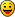Register

## Using equations to graph pictures on calculator

Discuss anything related to calculators. For specific help on certain games/programs check the Released Projects subforum.
Senior Member
Posts: 369
Joined: Tue Jan 03, 2012 11:24 pm
Calculators: Casio Afx 1.0, Casio fx-9860GII SD, Casio Classpad 330, Casio fx-CG20, Casio Classpad fx-CP400

### Using equations to graph pictures on calculator

A friend of mine started trying to draw pictures on the calculator only with equation types and math functions (ln, log, cos, tan, sin, abs...) available in casio calculators.

It's an interesting challenge. Many of the equations available in web to draw pictures are not compatible with casio calculators.

Below is the list of images made with equations until now (left: prizm; right: fx9860):

1 - Leaf

Author: A friend of mineEquations: Show
Y1 =Y2 =Y3 =Y4 =Y5 =Y6 =Y7 =Y8 =Y9 =Y10 =Y11 =Y12 =Y13 =Y14 =Y15 =Y16 =Y17 =2 - Heart

Author: Casio PolandEquations: Show
Y1 =Y2 =Y3 =Y4 =3 - Nike LogoEquations: Show
Y1 =Y2 =4 - Butterfly

Author: Niel KelceyEquations: Show
Y1 =Y2 =Y3 =Y4 =Y5 =Y6 =Y7 =Y8 =Y9 =Y10 =Y11 =5 - StarEquations: Show
Y1 =Y2 =Y3 =Y4 =Y5 =If you have more equations to graph pictures on calculator, you can share here.
SiO2 + CaCO3 ----------> CaSiO3 + CO2

Senior Member
Posts: 69
Joined: Sat Feb 02, 2013 4:29 am
Calculators: Casio Cfx Series, Casio fx-CG10

### Re: Using equations to graph pictures on calculator

This is very interesting and creative excersize.
However, if you remember that cubic splines are functions, you can take any artwork digitized as not too many splines and turn it into parametric polynomial plots.

Here is what I mean. To draw a cubic spline with control points (A,B), (C,D), (E,F), (G,H) set the folowing parametric plots:
"A(1-T)^3+3CT(1-T)^2+3ET^2(1-T)+GT^3"->Xt1
"B(1-T)^3+3DT(1-T)^2+3FT^2(1-T)+HT^3"->Yt1

Also, coefficients can be lists, so you can prefill several of them with control point coordinates and draw many splines using just one command. Unfortunately, i did not figure out how to make them all same color -- when taking coordinates from lists, the graphs system seems to rotate colors.

Another practical matter: you can use spline approximations of special curve to draw them. Like parabolas, ellipses, circular arcs -- which do not have their own casio basic routines.

Senior MemberPosts: 66
Joined: Sun Apr 15, 2012 4:00 pm
Calculators: Casio fx-9860G

### Re: Using equations to graph pictures on calculator

how did you do the interval thing on the 9860?

Senior Member
Posts: 369
Joined: Tue Jan 03, 2012 11:24 pm
Calculators: Casio Afx 1.0, Casio fx-9860GII SD, Casio Classpad 330, Casio fx-CG20, Casio Classpad fx-CP400

### Re: Using equations to graph pictures on calculator

First I write the equation:
Exemple: Y1=X

After, at the end of the equation I put a comma () and a parenthesis (+)

Now I have: Y1=X,[

I can write the first interval value:

Y1=X,[-5

before adding the second value I need to put another comma (), and only then I can write the second value:

Y1=X,[-5,5

to finish, I need to close parenthesis (+)

Done: Y1=X,[-5,5]
SiO2 + CaCO3 ----------> CaSiO3 + CO2

Senior Member
Posts: 69
Joined: Sat Feb 02, 2013 4:29 am
Calculators: Casio Cfx Series, Casio fx-CG10

### Re: Using equations to graph pictures on calculator

Amazing. Is it in the manual? I somehow missed it.
BTW, this domain specification works all the way back. I just tried it with 7700G and it is there.

Senior Member
Posts: 369
Joined: Tue Jan 03, 2012 11:24 pm
Calculators: Casio Afx 1.0, Casio fx-9860GII SD, Casio Classpad 330, Casio fx-CG20, Casio Classpad fx-CP400

### Re: Using equations to graph pictures on calculator

nsg wrote:Is it in the manual? I somehow missed it.

Maybe yes, but I learned this in class.SiO2 + CaCO3 ----------> CaSiO3 + CO2

Senior Member
Posts: 69
Joined: Sat Feb 02, 2013 4:29 am
Calculators: Casio Cfx Series, Casio fx-CG10

### Re: Using equations to graph pictures on calculator

Here is example of a program (fxCG) that draws family of splines using equation graph.

Code: Select all
`'ProgramMode:RUNParamType"A(1-T)^3+3C(1-T)_^<2>_T+3E(1-T)T_^<2>_+GT^3"->GraphXt1"B(1-T)^3+3D(1-T)_^<2>_T+3F(1-T)T_^<2>_+HT^3"->GraphYt10->TThetamin1->TThetamax0.05->TThetaptchG SelOff G SelOn 1ThinG 1For 0->I To 5 Step 0.5(-)6->A:(-)3->B:(-)3->C:6-I->D:0+I->E:(-)3->F:6->G:3->HDrawGraphNext`

Instead of assinging functions to graph memory, I tried to use Graph(X,Y)=( function which works great, except I do not know how to specify line styles for it.

Code: Select all
`'ProgramMode:RUN0->TThetamin1->TThetamax0.05->TThetaptchFor 0->I To 5 Step 0.5(-)6->A:(-)3->B:(-)3->C:6-I->D:0+I->E:(-)3->F:6->G:3->HGraph(X,Y)=(_A(1-T)^3+3C(1-T)_^<2>_T+3E(1-T)T_^<2>_+GT^3,B(1-T)^3+3D(1-T)_^<2>_T+3F(1-T)T_^<2>_+HT^3)Next`

Senior Member
Posts: 369
Joined: Tue Jan 03, 2012 11:24 pm
Calculators: Casio Afx 1.0, Casio fx-9860GII SD, Casio Classpad 330, Casio fx-CG20, Casio Classpad fx-CP400

### Re: Using equations to graph pictures on calculator

Graphing with white color:(and with a black .g3p behind).SiO2 + CaCO3 ----------> CaSiO3 + CO2